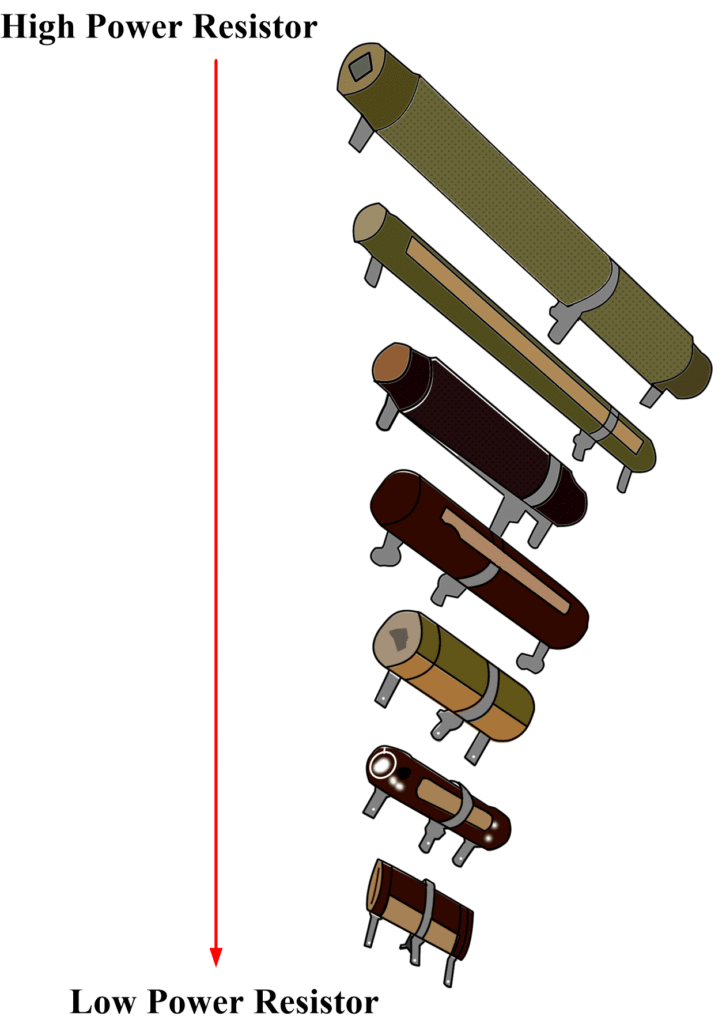# Resistor Power Rating | Power resistor

Want create site? Find Free WordPress Themes and plugins.

The physical size of a resistor is not determined by its resistance but by how much power, or heat, it can dissipate. It electric circuits, the unit of power is the watt (W), named in honor of James Watt.

One watt is the power dissipated when one ampere flows under one volt of applied emf.

Small resistors can dissipate or radiate less heat than larger ones because of their reduced surface area. If the temperature exceeds a specified value, the resistor may be damaged or may not return to its original resistance when allowed to cool.

## Resistor Power Rating

Because electrical operating conditions are so varied, resistors are made in a number of power ratings, ranging from 0.1 Watt to hundreds of Watts. Figure 1 shows several resistors in small wattage ratings.Fig.1: Carbon Composition Resistors arranged in their Wattage Ratings

Some wire-wound resistors of high power ratings are shown in figure 2.Fig.2: Types of Power Wire-Wound Resistors

The power rating applies to a resistor mounted in free space with adequate ventilation. In practice, these conditions are generally not met. Resistors are usually mounted under a chassis or on printed circuit boards, where the ventilation is restricted. It is good engineering practice, therefore, to at least double the calculated wattage rating to provide a margin of safety.

## Resistor Power Derating

When resistors are confined to restricted areas where the temperature significantly rises above ambient, the power rating must be de-rated, because the resistor cannot dissipate its rated power. A typical derating curve is shown in figure 3.Fig.3: Typical Power-Derating Curve

## Resistor Power Rating Example

A resistor rated at 0.5 W is to be operated at an ambient temperature of 100 oC. What is the maximum power the resistor can dissipate under these conditions?

### Solution

Refer to Figure 3, locate 100 oC on the x-axis. Project upward until you intersect the sloping part of the curve. Project horizontally to the left from this intercept to the y-axis. Read approximately 60 %, therefore

$0.5\text{ W * 0}\text{.6=0}\text{.3 W}$

So this is the maximum power that the resistor can dissipate under such conditions.

Did you find apk for android? You can find new Free Android Games and apps.